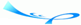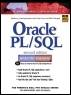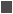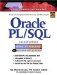# Lab 6.3 NULLIF and COALESCE Functions

 Team-FlyOracle® PL/SQL® Interactive Workbook, Second EditionBy Benjamin Rosenzweig, Elena Silvestrova Table of Contents Chapter 6.  Conditional Control: Case Statements

Lab Objectives

After this Lab, you will be able to:

• Use the NULLIF Function

• Use the COALESCE Function

The NULLIF and COALESCE functions are defined by the ANSI 1999 standard to be "CASE abbreviations." Both functions can be used as a variety of the CASE expression.

#### NULLIF Function

The NULLIF function compares two expressions. If they are equal, then the function returns NULL; otherwise, it returns the value of the first expression. The NULLIF has the following structure:

` NULLIF (expression1, expression2) `

If expression1 is equal to expression2, then NULLIF returns NULL. If expression1 does not equal expression2, NULLIF returns expression1. Note that the NULLIF function does the opposite of the NVL function. If the first expression is NULL, then NVL returns the second expression. If the first expression is not NULL, then NVL returns the first expression.

The NULLIF function is equivalent to the following CASE expression:

` CASE     WHEN expression1 = expression2 THEN NULL     ELSE expression1  END `

Consider the following example of NULLIF:FOR EXAMPLE

` DECLARE     v_num NUMBER := &sv_user_num;     v_remainder NUMBER;  BEGIN     -- calculate the remainder and if it is zero return a NULL     v_remainder := NULLIF(MOD(v_num,2),0);     DBMS_OUTPUT.PUT_LINE ('v_remainder: '||v_remainder);  END; `

This is example is somewhat similar to an example that you have seen earlier in this chapter. A value is assigned to the variable v_num at run-time. Next, this value is divided by 2, and its remainder is compared to 0 via the NULLIF function. If the remainder equals 0, the NULLIF function returns NULL; otherwise it returns the remainder. The value returned by the NULLIF function is stored in the variable v_remainder and displayed on the screen via the DBMS_OUTPUT.PUT_LINE statement. When run, the example produces the output shown below. For the first run, 5 is assigned to the variable v_num:

` Enter value for sv_user_num: 5  old   2:    v_num NUMBER := &sv_user_num;  new   2:    v_num NUMBER := 5;  v_remainder: 1  PL/SQL procedure successfully completed.`

For the second run, 4 is assigned to the variable v_num:

` Enter value for sv_user_num: 4  old   2:    v_num NUMBER := &sv_user_num;  new   2:    v_num NUMBER := 4;  v_remainder:  PL/SQL procedure successfully completed. `

In the first run, 5 is not divisible by 2, and the NULLIF function returns the value of the remainder. In the second run, 4 is divisible by 2, and the NULLIF function returns NULL as the value of the remainder.

The NULLIF function has a restriction: You cannot assign a literal NULL to expression1. You learned about literals in Chapter 3. Consider another output produced by the preceding example. For this run, the variable v_num is assigned NULL:

` Enter value for sv_user_num: NULL  old   2:    v_num NUMBER := &sv_user_num;  new   2:    v_num NUMBER := NULL;  v_remainder:  PL/SQL procedure successfully completed. `

When NULL is assigned to the variable v_num, both the MOD and NULLIF functions return NULL. This example does not produce any errors because the literal NULL is assigned to the variable v_num, and it is not used as the first expression of the NULLIF function. Next, consider this modified version of the preceding example:FOR EXAMPLE

` DECLARE     v_remainder NUMBER;  BEGIN     -- calculate the remainder and if it is zero return a     NULL     v_remainder := NULLIF(NULL,0);     DBMS_OUTPUT.PUT_LINE ('v_remainder: '||v_remainder);  END; `

In the previous version of this example, the MOD function is used as expression1. In this version, the literal NULL is used in place of the MOD function, and as a result, this example produces the following syntax error:

`    v_remainder := NULLIF(NULL,0);                           *  ERROR at line 5:  ORA-06550: line 5, column 26:  PLS-00619: the first operand in the NULLIF expression must  not be NULL  ORA-06550: line 5, column 4:  PL/SQL: Statement ignored `

#### COALESCE Function

The COALESCE function compares each expression to NULL from the list of expressions and returns the value of the first non-null expression. The COALESCE function has the following structure:

` COALESCE (expression1, expression2, …, expressionN) `

If expression1 evaluates to NULL, then expression2 is evaluated. If expression2 does not evaluate to NULL, then the function returns expression2. If expression2 also evaluates to NULL, then the next expression is evaluated. If all expressions evaluate to NULL, the function returns NULL.

Note that the COALESCE function is like a nested NVL function:

` NVL(expression1, NVL(expression2, NVL(expression3,…))) `

The COALESCE function can also be used as an alternative to a CASE expression. For example,

` COALESCE (expression1, expression2) `

is equivalent to

` CASE     WHEN expression1 IS NOT NULL THEN expression1     ELSE expression2  END `

If there are more than two expressions to evaluate, then

` COALESCE (expression1, expression2, …, expressionN) `

is equivalent to

` CASE     WHEN expression1 IS NOT NULL THEN expression1     ELSE COALESCE (expression2, …, expressionN)  END `

Consider the following example of the COALESCE function:FOR EXAMPLE

` SELECT e.student_id, e.section_id, e.final_grade,         g.numeric_grade,         COALESCE(e.final_grade, g.numeric_grade, 0) grade    FROM enrollment e, grade g   WHERE e.student_id = g.student_id     AND e.section_id = g.section_id     AND e.student_id = 102     AND g.grade_type_code = 'FI'; `

This SELECT statement returns the following output:

` STUDENT_ID SECTION_ID FINAL_GRADE NUMERIC_GRADE      GRADE  ---------- ---------- ----------- ------------- ----------        102         86                        85         85         102         89          92            92         92 `

The value of GRADE equals the value of the NUMERIC_GRADE in the first row. The COALESCE function compares the value of the FINAL_GRADE to NULL. If it is NULL, then the value of the NUMERIC_GRADE is compared to NULL. Because the value of the NUMERIC_GRADE is not NULL, the COALESCE function returns the value of the NUMERIC_GRADE. The value of GRADE equals the value of FINAL_GRADE in the second row. The COALESCE function returns the value of FINAL_GRADE because it is not NULL.

The COALESCE function shown in the previous example is equivalent to the following NVL statement and CASE expression:

` NVL(e.final_grade, NVL(g.numeric_grade, 0))  CASE     WHEN e.final_grade IS NOT NULL THEN e.final_grade     ELSE COALESCE(g.numeric_grade, 0)  END `

The COALESCE function has the following restriction: At least one of its expressions must not contain a literal NULL. Consider the following example and its output:FOR EXAMPLE

` SELECT COALESCE(NULL, 3, 8)    FROM DUAL;  COALESCE(NULL,3,8)  ------------------                  3 `

Next, consider this modified version of the same SELECT statement and the syntax error it generatesFOR EXAMPLE

` SELECT COALESCE(NULL, NULL, NULL)    FROM DUAL;  SELECT COALESCE(NULL, NULL, NULL)         *  ERROR at line 1:  ORA-00938: not enough arguments for function `

The SELECT statement causes a syntax error because all of the expressions in the COALESCE function contain the literal NULL.

#### Lab 6.3 Exercises

##### 6.3.1 Use the NULLIF Function

In this exercise, you will modify the following script. Instead of using the searched CASE expression, you will use the NULLIF function. Note that the SELECT INTO statement uses ANSI 1999 SQL standard.You will find detailed explanations and examples of the statements using new ANSI 1999 SQL standard in Appendix E and in Oracle help. Throughout this book we try to provide you with examples illustrating both standards; however our main focus is on PL/SQL features rather than SQL.

` -- ch06_4a.sql, version 1.0  SET SERVEROUTPUT ON  DECLARE     v_final_grade NUMBER;  BEGIN     SELECT CASE               WHEN e.final_grade = g.numeric_grade THEN NULL               ELSE g.numeric_grade            END       INTO v_final_grade       FROM enrollment e       JOIN grade g         ON (e.student_id = g.student_id         AND e.section_id = g.section_id)      WHERE e.student_id = 102        AND e.section_id = 86        AND g.grade_type_code = 'FI';     DBMS_OUTPUT.PUT_LINE ('Final grade: '||v_final_grade);  END; `

In the preceding script, the value of the final grade is compared to the value of the numeric grade. If these values are equal, the CASE expression returns NULL. In the opposite case, the CASE expression returns the numeric grade. The result of the CASE expression is then displayed on the screen via the DBMS_OUTPUT.PUT_LINE statement.

Answer the following questions:

 a) Modify script ch06_4a.sql. Substitute the CASE expression with the NULLIF function. b) Run the modified version of the script and explain the output produced. c) Change the order of columns in the NULLIF function. Run the modified version of the script and explain the output produced.

##### 6.3.2 Use the COALESCE Function

In this exercise, you will modify the following script. Instead of using the searched CASE expression, you will use the COALESCE function.

` -- ch06_5a.sql, version 1.0  SET SERVEROUTPUT ON  DECLARE     v_num1 NUMBER := &sv_num1;     v_num2 NUMBER := &sv_num2;     v_num3 NUMBER := &sv_num3;     v_result NUMBER;  BEGIN     v_result := CASE                    WHEN v_num1 IS NOT NULL THEN v_num1                    ELSE                       CASE                          WHEN v_num2 IS NOT NULL THEN v_num2                          ELSE v_num3                       END                    END;     DBMS_OUTPUT.PUT_LINE ('Result: '||v_result);  END; `

In the preceding script, the list consisting of three numbers is evaluated as follows: If the value of the first number is not NULL, then the outer CASE expression returns the value of the first number. Otherwise, control is passed to the inner CASE expression, which evaluates the second number. If the value of the second number is not NULL, then the inner CASE expression returns the value of the second number; in the opposite case, it returns the value of the third number.

The preceding CASE expression is equivalent to the following two CASE expressions:

` CASE     WHEN v_num1 IS NOT NULL THEN v_num1     WHEN v_num2 IS NOT NULL THEN v_num2     ELSE v_num3  END  CASE     WHEN v_num1 IS NOT NULL THEN v_num1     ELSE COALESCE(v_num2, v_num3)  END `

Answer the following questions:

 a) Modify script ch06_5a.sql. Substitute the CASE expression with the COALESCE function. b) Run the modified version of the script and explain the output produced. Use the following values for the list of numbers: NULL, 1, 2. c) What output will be produced by the modified version of the script if NULL is provided for all three numbers? Try to explain your answer before you run the script.

#### Lab 6.3 Exercise Answers

This section gives you some suggested answers to the questions in Lab 6.3, with discussion related to how those answers resulted. The most important thing to realize is whether your answer works. You should figure out the implications of the answers here and what the effects are from any different answers you may come up with.

##### 6.3.1 Answers

 a) Modify script ch06_4a.sql. Substitute the CASE expression with the NULLIF function. A1: Answer: Your script should look similar to the following script. Changes are shown in bold letters.` -- ch06_4b.sql, version 2.0 SET SERVEROUTPUT ON DECLARE v_final_grade NUMBER; BEGIN SELECT NULLIF(g.numeric_grade, e.final_grade) INTO v_final_grade FROM enrollment e JOIN grade g ON (e.student_id = g.student_id AND e.section_id = g.section_id) WHERE e.student_id = 102 AND e.section_id = 86 AND g.grade_type_code = 'FI'; DBMS_OUTPUT.PUT_LINE ('Final grade: '||v_final_grade); END; `In the original version of the script, you used CASE expression in order to assign a value to the variable v_final_grade as follows:` CASE WHEN e.final_grade = g.numeric_grade THEN NULL ELSE g.numeric_grade END `The value stored in the column FINAL_GRADE is compared to the value stored in the column NUMERIC_GRADE. If these values are equal, then NULL is assigned to the variable v_final_grade; otherwise, the value stored in the column NUMERIC_GRADE is assigned to the variable v_letter_grade.In the new version of the script you substitute the CASE expression with the NULLIF function as follows:` NULLIF(g.numeric_grade, e.final_grade) `It is important to note that the NUMERIC_GRADE column is referenced first in the NULLIF function. You will recall that the NULLIF function compares expression1 to expression2. If expression1 equals expression2, the NULLIF functions returns NULL. If expression1 does not equal expression2, the NULLIF function returns expression1. In order to return the value stored in the column NUMERIC_GRADE, you must reference it first in the NULLIF function. b) Run the modified version of the script and explain the output produced. A2: Answer: Your output should look similar to the following:` Final grade: 85 PL/SQL procedure successfully completed. `The NULLIF function compares values stored in the columns NUMERIC_GRADE and FINAL_GRADE. Because the column FINAL_GRADE is not populated, the NULLIF function returns the value stored in the column NUMERIC_GRADE. This value is assigned to the variable v_final_grade and displayed on the screen with the help of the DBMS_OUTPUT.PUT_LINE statement. c) Change the order of columns in the NULLIF function. Run the modified version of the script and explain the output produced. A3: Answer: Your script should look similar to the following. Changes are shown in bold letters.` -- ch06_4c.sql, version 3.0 SET SERVEROUTPUT ON DECLARE v_final_grade NUMBER; BEGIN SELECT NULLIF(e.final_grade, g.numeric_grade) INTO v_final_grade FROM enrollment e JOIN grade g ON (e.student_id = g.student_id AND e.section_id = g.section_id) WHERE e.student_id = 102 AND e.section_id = 86 AND g.grade_type_code = 'FI'; DBMS_OUTPUT.PUT_LINE ('Final grade: '||v_final_grade); END; `The example produces the following output:` Final grade: PL/SQL procedure successfully completed. `In this version of the script, the columns NUMERIC_GRADE and FINAL_GRADE are listed in the opposite order as follows:` NULLIF(e.final_grade, g.numeric_grade) `The value stored in the column FINAL_GRADE is compared to the value stored in the column NUMERIC_GRADE. Because these values are not equal, the NULLIF function returns the value of the column FINAL_GRADE. This column is not populated, so NULL is assigned to the variable v_final_grade.

##### 6.3.2 Answers

 a) Modify script ch06_5a.sql. Substitute the CASE expression with the COALESCE function. A1: Answer: Your script should look similar to the following script. Changes are shown in bold letters.` -- ch06_5b.sql, version 2.0 SET SERVEROUTPUT ON DECLARE v_num1 NUMBER := &sv_num1; v_num2 NUMBER := &sv_num2; v_num3 NUMBER := &sv_num3; v_result NUMBER; BEGIN v_result := COALESCE(v_num1, v_num2, v_num3); DBMS_OUTPUT.PUT_LINE ('Result: '||v_result); END; `In the original version of the script you used nested CASE expression in order to assign a value to the variable v_result as follows:` CASE WHEN v_num1 IS NOT NULL THEN v_num1 ELSE CASE WHEN v_num2 IS NOT NULL THEN v_num2 ELSE v_num3 END END; `In the new version of the script you substitute the CASE expression with the COALESCE function as follows:` COALESCE(v_num1, v_num2, v_num3) `Based on the values stored in the variables v_num1, v_num2, and v_num3, the COALESCE function returns the first non-null variable. b) Run the modified version of the script and explain the output produced. Use the following values for the list of numbers: NULL, 1, 2. A2: Answer: Your output should look similar to the following:` Enter value for sv_num1: null old 2: v_num1 NUMBER := &sv_num1; new 2: v_num1 NUMBER := null; Enter value for sv_num2: 1 old 3: v_num2 NUMBER := &sv_num2; new 3: v_num2 NUMBER := 1; Enter value for sv_num3: 2 old 4: v_num3 NUMBER := &sv_num3; new 4: v_num3 NUMBER := 2; Result: 1 PL/SQL procedure successfully completed. `The COALESCE function evaluates its expressions in the sequential order. The variable v_num1 is evaluated first. Because the variable v_num1 is NULL, the COALESCE function evaluates the variable v_num2 next. Because the variable v_num2 is not NULL, the COALSECE function returns the value of the variable v_num2. This value is assigned to the variable v_result and is displayed on the screen via DBMS_ OUTPUT.PUT_LINE statement. c) What output will be produced by the modified version of the script if NULL is provided for all three numbers? Try to explain your answer before you run the script. A3: Answer: The variables v_num1, v_num2, and v_num3 are evaluated in the sequential order by the COALESCE function. When NULL is assigned to these variables, none of the evaluations produce a non-null result. So the COALESCE function returns NULL when all expressions evaluate to NULL.Your output should look similar to the following:` Enter value for sv_num1: null old 2: v_num1 NUMBER := &sv_num1; new 2: v_num1 NUMBER := null; Enter value for sv_num2: null old 3: v_num2 NUMBER := &sv_num2; new 3: v_num2 NUMBER := null; Enter value for sv_num3: null old 4: v_num3 NUMBER := &sv_num3; new 4: v_num3 NUMBER := null; Result: PL/SQL procedure successfully completed. `

#### Lab 6.3 Self-Review Questions

In order to test your progress, you should be able to answer the following questions.

Answers appear in Appendix A, Section 6.3.

 1) A NULLIF function returns NULL if _____ expression1 equals expression2._____ expression1 does not equal expression2. 2) A NULLIF function is just like NVL function. _____ True_____ False 3) You can specify literal NULL in the first expression of the NULLIF function. _____ True_____ False 4) A COALESCE function returns _____ first null expression._____ first non-null expression._____ first expression only. 5) You can never specify literal NULL as one of the expressions in the COALESCE function. _____ True_____ False

 Team-FlyTopOracle PL/SQL Interactive Workbook (2nd Edition)
ISBN: 0130473200
EAN: 2147483647
Year: 2002
Pages: 146

Similar book on Amazon

flylib.com © 2008-2017.
If you may any questions please contact us: flylib@qtcs.net